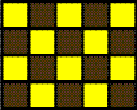A NOTE ON CHESSBOARD MATRICES

Paulus Gerdes

Mozambican Ethnomathematics Research Centre, C.P. 915,
Maputo, Mozambique
pgerdes@virconn.com

Abstract:

The paper introduces the concepts of positive and negative chessboard matrices and presents the properties of the multiplication of chessboard matrices.

A normal chessboard has dimensions 8×8. Its top left chequer (A8) is white. We may define a generalised chessboard of dimensions m×n as a m×n chequer board where the black (dark coloured) and white (light coloured) chequers alternate, being the top left chequer white. Figure 1 displays the chessboard of dimensions 4×5.Chessboard of dimensions 4×5

Figure 1

Consider a matrix inscribed in a chessboard. If all the matrix elements on the black chequers are 0, we will call the matrix a positive chessboard matrix. If all the matrix elements on the white chequers are 0, we will call the matrix a negative chessboard matrix. In other words, a matrix A of dimensions m×n is a positive chessboard matrix if

a(i,j) = 0 for i+j = odd (i = 1, , m; j = 1, , n).

And a matrix A of dimensions m×n is a negative chessboard matrix if

a(i,j) = 0 for i+j = even (i = 1, , m; j = 1, , n).

Figure 2 presents examples of positve and negative chessboard matrices of dimensions 4×5 (P and N) and 5×7 (P and N), respectively.

 3 0 -1 0 4 0 3 0 1 0 0 2 0 5 0 1 0 6 0 4 1 0 3 0 2 0 2 0 -3 0 0 4 0 -2 0 4 0 5 0 2 P N
 2 0 3 0 -2 0 5 0 2 0 1 0 5 0 0 -1 0 1 0 4 0 -1 0 3 0 7 0 -4 -2 0 4 0 2 0 8 0 3 0 2 0 -3 0 0 2 0 -3 0 6 0 2 0 -2 0 4 0 1 5 0 4 0 1 0 2 0 4 0 1 0 3 0 P N

Figure 2

Their products (PP, PN, NP and NN) are displayed in Figure 3. Immediately it may be noted that the products are positive if both matrices have the same sign and are negative if both matrices have opposite signs.

 28 0 21 0 -4 0 15 0 19 0 5 0 30 0 0 8 0 -13 0 38 0 8 0 -4 0 34 0 -3 6 0 23 0 6 0 33 0 19 0 9 0 2 0 0 -8 0 10 0 4 0 -8 0 16 0 20 0 -18 PP PN 0 -1 0 0 0 18 0 -1 0 7 0 25 0 -11 10 0 43 0 14 0 61 0 36 0 17 0 -1 0 0 -8 0 11 0 -10 0 -8 0 12 0 2 0 -11 8 0 40 0 4 0 64 0 31 0 16 0 11 0 NP NN

Figure 3

The following theorem can be easily proven:

Theorem: The multiplication of positive and negative chessboard matrices satifies the following multiplication table:
 A B AB + + + + - - - + - - - +

The multiplication table is the same as the multiplication tables for positive and negative numbers, for even and odd numbers, for positive (second order) and negative (first order) cycle matrices of period p (see Gerdes 2002a), for positive and negative helix matrices of period p (see Gerdes 2002b), and for positive and negative cylinder matrices of period p (Gerdes 2002c). Chessboard matrices of dimensions (2m)×(2n) constitute a particular class of cylinder matrices of period 2mn (see the example in Figure 4).

 0 1 0 2 0 -1 3 0 4 0 -3 0 0 -2 0 7 0 4 5 0 6 0 1 0

Figure 4

References:

Gerdes, Paulus (2002a), From Liki-designs to cycle matrices: The discovery of attractive new symmetries, Visual Mathematics, Vol. 4, No. 1, March 2002 (http://members.tripod.com/vismath7/gerd/)

Gerdes, Paulus (2002b), Helix matrices, Visual Mathematics, Vol. 4, No. 2, June 2002 (http:/members.tripod.com/vismath8/gerdhel/hel.htm)

Gerdes, Paulus (2002c), Cylinder matrices, Visual Mathematics, Vol. 4, No. 2, June 2002 (http:/members.tripod.com/vismath8/gerdcyl/cyl1.htm)